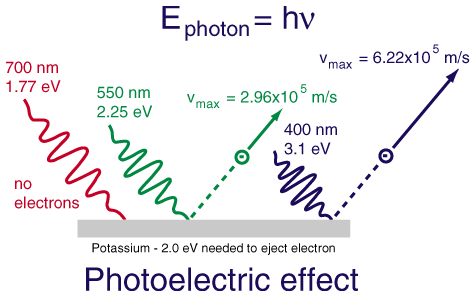# Quantum theory 1: The dual nature of light

The wave nature of light:

One way of looking at light is as an electromagnetic wave, consisting of perpendicular, oscillating electric and magnetic fields.We characterise it by is wavelength, (and therefore its frequency). The wavelength, (λ) is the distance between two waves, and the frequency (ν) is the number of waves that pass through a point per unit time.The wavelength and frequency are related to one another by c, the speed of light:

c = λν

The range of electromagnetic radiation is called the electromagnetic spectrum, (shown below) and ranges from wavelengths smaller than atoms (gamma rays) to kilometres (radio waves). Visible light makes up a very small section, ranging from about 400 nm (violet) to 700 nm (red).There is significant evidence for the wave nature of light – we see diffraction effects, it can be polarised and it can interfere with itself.

The particle nature of light:

Isaac Newton was a big proponent of the particle, (or, in his words “corpuscular”) theory of light, but this view was largely abandoned in favour of the wave model until the beginning of the 20th century. Einstein won his Nobel prize for his paper on the photoelectric effect which showed the energy in light was “quantised”, based on the work of Max Planck.

Planck showed that there was a close relationship between the light emitted by a solid and its temperature. A material will glow “white hot” at 1200°C, but red at 750°C. He found that, when using Boltzmann’s statistical version of the second law of thermodynamics, the energy emitted by a solid could only be E = nhν, where n is an integer value – ie, the energy is quantised. The value h is Planck’s constant and equal to 6.63 x 10-34 J.s.

Einstein took this idea and showed that it applied to the photoelectric effect. This was an experiment where light is shone on the clean surface of a metal and the electrons released are measured. Electrons are ejected from an atom when enough energy is absorbed; however this only occurs when light above a certain frequency is used. This threshold frequency is characteristic of each metal.To explain this, the electron must be ejected using the energy from a single photon, and that photon must have greater energy than the ionisation energy of the atom. If the photon doesn’t have enough energy, no matter how many photons hit the metal, the electron won’t be ejected. So, light must be quantised and behave as a particle!

How do we reconcile these two behaviours? Not in any really satisfying way. We say that light behaves as both a wave and a particle, (known as the wave-particle duality).

http://hyperphysics.phy-astr.gsu.edu/hbase/mod1.html

http://en.wikipedia.org/wiki/Wave%E2%80%93particle_duality